# SAT Math Multiple Choice Question 803: Answer and Explanation

### Test Information

Question: 803

8. If 10a = 6b + 7 and a – 6b = 34, then what is the value of –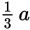?

• A. –1
• B. 1
• C.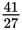• D.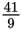Explanation:

B

Difficulty: Medium

Category: Heart of Algebra / Systems of Linear Equations

Strategic Advice: Sometimes, conventional substitution will get you to the answer, but there may be a faster (but cleverly hidden) alternate route. The clue in this question is the presence of 6b in both equations.

Getting to the Answer: With a little rearranging, you can isolate a - 6b in the first equation, substitute 34 (from the second equation), and end up with only one equation and one variable. Start by moving 6b to the left side, and then rewrite 10a as 9a + a. Once there, you can substitute and solve for a: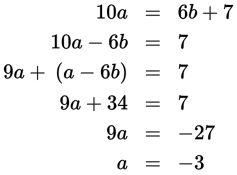You're asked to find -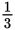a, so multiply - 3 by -to get 1, making (B) the correct answer.